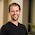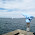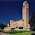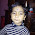# The 20% Statistician

A blog on statistics, methods, philosophy of science, and open science. Understanding 20% of statistics will improve 80% of your inferences.

## Sunday, December 18, 2016

### Why Type 1 errors are more important than Type 2 errors (if you care about evidence)

After performing a study, you can correctly conclude there is an effect or not, but you can also incorrectly conclude there is an effect (a false positive, alpha, or Type 1 error) or incorrectly conclude there is no effect (a false negative, beta, or Type 2 error).

The goal of collecting data is to provide evidence for or against a hypothesis. Take a moment to think about what ‘evidence’ is – most researchers I ask can’t come up with a good answer. For example, researchers sometimes think p-values are evidence, but p-values are only correlated with evidence.

Evidence in science is necessarily relative. When data is more likely assuming one model is true (e.g., a null model) compared to another model (e.g., the alternative model), we can say the model provides evidence for the null compared to the alternative hypothesis. P-values only give you the probability of the data under one model – what you need for evidence is the relative likelihood of two models.

Bayesian and likelihood approaches should be used when you want to talk about evidence, and here I’ll use a very simplistic likelihood model where we compare the relative likelihood of a significant result when the null hypothesis is true (i.e., making a Type 1 error) with the relative likelihood of a significant result when the alternative hypothesis is true (i.e., *not* making a Type 2 error).

Let’s assume we have a ‘methodological fetishist’ (Ellemers, 2013) who is adamant about controlling their alpha level at 5%, and who observes a significant result. Let’s further assume this person performed a study with 80% power, and that the null hypothesis and alternative hypothesis are equally (50%) likely. The outcome of the study has a 2.5% probability of being a false positive (a 50% probability that the null hypothesis is true, multiplied by a 5% probability of a Type 1 error), and a 40% probability of being a true positive (a 50% probability that the alternative hypothesis is true, multiplied by an 80% probability of finding a significant effect).

The relative evidence for H1 versus H0 is 0.40/0.025 = 16. In other words, based on the observed data, and a model for the null and a model for the alternative hypothesis, it is 16 times more likely that the alternative hypothesis is true than that the null hypothesis is true. For educational purposes, this is fine – for statistical analyses, you would use formal likelihood or Bayesian analyses.

Now let’s assume you agree that providing evidence is a very important reason for collecting data in an empirical science (another goal of data collection is estimation – but I’ll focus on hypothesis testing here). We can now ask ourselves what the effect of changing the Type 1 error or the Type 2 error (1-power) is on the strength of our evidence. And let’s agree that we will conclude that whichever error impacts the strength of our evidence the most, is the most important error to control. Deal?

We can plot the relative likelihood (the probability a significant result is a true positive, compared to a false positive) assuming H0 and H1 are equally likely, for all levels of power, and for all alpha levels. If we do this, we get the plot below:

Or for a rotating version (yeah, I know, I am an R nerd):

So when is the evidence in our data the strongest? Not surprisingly, this happens when both types of errors are low: the alpha level is low, and the power is high (or the Type 2 error rate is low). That is why statisticians recommend low alpha levels and high power. Note that the shape of the plot remains the same regardless of the relative likelihood H1 or H0 is true, but when H1 and H0 are not equally likely (e.g., H0 is 90% likely to be true, and H1 is 10% likely to be true) the scale on the likelihood ratio axis increases or decreases.

Now for the main point in this blog post: we can see that an increase in the Type 2 error rate (or a reduction in power) reduces the evidence in our data, but it does so relatively slowly. However, we can also see that an increase in the Type 1 error rate (e.g., as a consequence of multiple comparisons without controlling for the Type 1 error rate) quickly reduces the evidence in our data. Royall (1997) recommends that likelihood ratios of 8 or higher provide moderate evidence, and likelihood ratios of 32 or higher provide strong evidence. Below 8, the evidence is weak and not very convincing.

If we calculate the likelihood ratio for alpha = 0.05, and power from 1 to 0.1 in steps of 0.1, we get the following likelihood ratios: 20, 18, 16, 14, 12, 10, 8, 6, 4, 2. With 80% power, we get the likelihood ratio of 16 we calculated above, but even 40% power leaves us with a likelihood ratio of 8, or moderate evidence (see the figure above). If we calculate the likelihood ratio for power = 0.8 and alpha levels from 0.05 to 0.5 in steps of 0.05, we get the following likelihood ratios: 16, 8, 5.3, 4, 3.2, 2.67, 2.29, ,2, 1.78, 1.6. An alpha level of 0.1 still yields moderate evidence (assuming power is high enough!) but further inflation makes the evidence in the study very weak.

To conclude: Type 1 error rate inflation quickly destroys the evidence in your data, whereas Type 2 error inflation does so less severely.

Type 1 error control is important if we care about evidence. Although I agree with Fiedler, Kutzner, and Kreuger (2012) that a Type 2 error is also very important to prevent, you simply can not ignore Type 1 error control if you care about evidence. Type 1 error control is more important than Type 2 error control, because inflating Type 1 errors will very quickly leave you with evidence that is too weak to be convincing support for your hypothesis, while inflating Type 2 errors will do so more slowly. By all means, control Type 2 errors - but not at the expense of Type 1 errors.

I want to end by pointing out that Type 1 and Type 2 error control is not a matter of ‘either-or’. Mediocre statistics textbooks like to point out that controlling the alpha level (or Type 1 error rate) comes at the expense of the beta (Type 2) error, and vice-versa, sometimes using the horrible seesaw metaphor below:

But this is only true if the sample size is fixed. If you want to reduce both errors, you simply need to increase your sample size, and you can make Type 1 errors and Type 2 errors are small as you want, and contribute extremely strong evidence when you collect data.

Ellemers, N. (2013). Connecting the dots: Mobilizing theory to reveal the big picture in social psychology (and why we should do this): The big picture in social psychology. European Journal of Social Psychology, 43(1), 1–8. https://doi.org/10.1002/ejsp.1932
Fiedler, K., Kutzner, F., & Krueger, J. I. (2012). The Long Way From -Error Control to Validity Proper: Problems With a Short-Sighted False-Positive Debate. Perspectives on Psychological Science, 7(6), 661–669. https://doi.org/10.1177/1745691612462587
Royall, R. (1997). Statistical Evidence: A Likelihood Paradigm. London ; New York: Chapman and Hall/CRC.

#### 32 comments:

1.I always enjoy your posts! The code, the graphs, all of it!

I have a quick question (I hope it's quick!):
Can you please explain a little more what it means to say there's an 80% probability of rejecting a false null alongside a 50% probability that the null is true?

2."P-values only give you the probability of one model"...i thought o values provide a probability of obtaining data. Isn't what you're claiming a 'fallacy of the transposed conditional'.

1.added 'data under'

3.Really interesting (and I appreciate the educational rather than mathematical approach). Does the conclusion hold up for a wide range of likelihoods of alernative probabilities?

1.alternative probabilities of what?

4.I've never really understood claims about "controlling the Type I error rate." The significance level alpha is chosen before the test is run. So if alpha is, say, 0.05, then the Type I error rate is always 5% no matter the sample size. A related issue is that it only makes sense to talk about Type I errors under the assumption that the null model is "true", but of course in reality, the null is never actually, literally true. (This is one reason I prefer Gelman's formulation of Type S and Type M errors.)

1.Please read my blog post about whether the null is never true (http://daniellakens.blogspot.nl/2014/06/the-null-is-always-false-except-when-it.html). Your statement: "So if alpha is, say, 0.05, then the Type I error rate is always 5% no matter the sample size." is incorrect.

2.Your statement that his statement is incorrect is incorrect. The probability of a type I error is a conditional probability (conditional on the null being true). Suppose that under the null your test statistic follows a standard normal distribution, and suppose you decide on alpha = .05. So, you decide to reject the null only if the absolute value of the statistic exceeds 1.96, because if the null is true using this criterion leads to 5% incorrect rejections. (Type 1 errors). Note that irregardless of sample size, 95% of values of your statistic will be between (approximately) -1.96 and 1.96. Or in terms of p-values: with alpha = .05, you will reject when p <= .05. Now, the distribution of the p-value is uniform if the null-hypothesis is true (independent of sample size), so the probability of p < .05 is .05 irregardless of sample size and the probability of a type I error is 5% irregardless of sample size.

3.Hi Gerben, you need to to take prior probabilities into account. If you do 1000 studies, and the null is true in 500 and there is an effect in 500, the Type 1 error rate is 2.5%. See week 3 in my MOOC for a more extensive explanation.

4.Thanks for your reply.
The false positive rate (FPR) is not the same as the type I error rate: FPR = the probability that you reject AND the null-hypothesis is true (=alpha * Base Rate) or in your terms (alpha * prior probability), but the type I error rate is the probability that you reject GIVEN the null-hypothesis is true: (alpha * Base Rate) / Base Rate = alpha.
Alpha is independent of the base rate and independent of sample size, and you seem to claim that alpha is not independent of sample size.

5.The FPR is a ratio of significant results only, not of all results. The Type 1 error rate is based on all results. Again, feel free to follow my MOOC week 3 to learn more about this, it would save me some time.

6.Will do. (I know my comment is not really about the point you are trying to make in your post, so let's leave it at this. But still very curious about how sample size is related to type I error as defined by Newman and Pearson).

7.Oops neyman...

8.As explained in my MOOC, Type 1 error rate is independent of the sample size.

9.Indeed, and that's what Sean also said. So, now things get really confusing:
First you say (in your reply to Sean): "Your statement: "So if alpha is, say, 0.05, then the Type I error rate is always 5% no matter the sample size." is incorrect." But now you say (in a reply to me): "As explained in my MOOC, Type 1 error rate is independent of the sample size". I care about "truth" (maybe as much as you seem to care about "evidence"), but certainly both of your replies cannot be true at the same time.

10.It was the 5% that was incorrect, not the part about the sample. Due to time constraints, this is my last reply about this.

11.Thanks for your time and replies Daniel.

5.Hi Daniel,
very interesting! I actually thought about p-values and how they change H0/H1 probability a lot lately, therefore, this comes to a perfect time!
Next time a post on the relation of pvals to BFs maybe? :)

1.Hm, I just re-skimmed your post "The relation between p-values and the probability H0 is true is not weak enough to ban p-values". There you stated that "Lower p-values always mean P(H0|D) is lower, compared to higher p-values." Isn't that a form of absolute evidence? I mean, pvals are still only related to evidence because the p postulate (same p value, same evidence) does not hold. but why is that not a form of absolute evidence (if pvals would be evidence)?

2.no, because you also need P(H1|D)

6.Very interesting and well done post - thank you. Question - does the relative implication of the risk/benefit of a Type 1 or 2 error matter in deciding which is most important to control? Example - assume we are testing if Drug A cures cancer. Type 1 error rate is very important to control. If we make a Type 1 error, we subject people to side effects of Drug A for no benefit, etc. Now, consider testing if Supplement B improves joint pain. B is very cheap, over the counter, and no side effects. A Type 1 error doesn't "cost" much, little money, no side effects, etc. A Type 2 error, though, would remove a cost effective pain control for suffering people. In this case, would an alpha of 0.1 or even 0.2 be acceptable? Assume Supplement B has no big marketing department and therefore we can only run a small study.

1.Sure - but my post is about evidence.

7.This is an interesting idea, and it gave me a good “think,” thanks for that! However, I think that the “horrible seesaw methaphor” has some value here because researcher may not always be in the situation where alpha and power are truly independent. From that perspective, it would also be necessary to take the frequency of positive findings into account if the source of evidence is indeed considered a positive finding.

1) Regarding alpha and power

Essentially, the question in this post can be reformulated as “What do I gain from a lower alpha if the power remains the same?” (and vice versa, since the two are treated independently). However, alpha is actually a determinant of the power of a test, and the two can only be treated independently if the sample size, etc. are allowed to vary. This is the situation we have when we plan experiments, and the message of the post is a very good one (as I understand): Given a certain range in sample size that may be feasible, it is best to choose the lowest possible alpha (i.e., maximum feasible sample size) that doesn't reduce power (or not that much).

However, I think the opposite perspective is also important: When we have already conducted an experiment, that is, the sample size and effect size are already fixed. In that case, alpha and power are related. The lower alpha (stricter test) the lower the power.

I made a graph that illustrates this (here: https://pbs.twimg.com/media/C0DYs44W8AEQge2.jpg). To the left there is the intial finding. Evidence increases as alpha increases (as long as power remains constant). In the middle, I assumed that there is a sample (n=25, d=0.5, sd=1) and I took into account that the power becomes lower in such a case when alpha is adjusted. The basic finding is the same, but the curve is a bit less steep.

(2) Frequency

There is another “puzzle piece” I would like to throw into the ring, though. In the post, evidence is defined as the ratio of the probabilities of true vs. false positives, and as such, it relies on the fact that we can actually observe a positive finding. However, if the power drops as alpha decreases (again: with sample fixed from an already conducted experiment), it also becomes less likely that we can observe positive finding.

In other words, this perspective can be summarized as “If we imagine a series of (fixed) experiments, do we gain anything if we conduct stricter tests (at lower alpha)?” In the end, this question has to consider two points: (1) the evidence provided by positive findings and (2) the frequency of positive findings. Both is affected by alpha, and if we correct for (i.e., multiply with) the probability of observing a positive finding, there emerges a different picture.
This shown in the right graph (here: https://pbs.twimg.com/media/C0DYs44W8AEQge2.jpg). Here the (average) evidence accumulated by positive findings becomes lower again with very low alphas. It peaks in this case around 2%. I gave the code below. Feel free to play with it. Interestingly, quite low values of alpha seem to be “optimal” from that perspective when the power is relatively high (e.g., large samples or large effect size).

What this means is that: If we consider alpha and power separate, then alpha takes the cake. But this leaves the sample size needed to conduct such experiments open (and it may be very expensive to do so). If we ask the question differently: “What if I already have a sample? Can a lower alpha help me now?”, then the answer probably is: “It depends.”

Again, nice post. I hope the additional perspective is useful. I think that both perspectives essentially lead to the same conclusion, which is: The larger the sample, the better :)

8.Code adapted from the original post (note that I used conditional probabilities for the LRs, but that doesn't have any consequence here):

# ** hyperparameters
delta <- 0.5
sd <- 1
n <- 25
pH0 <- 0.5

# plot
prec <- 5 # plotting precision
png("LR_alpha.png", width=1200, height=400, pointsize=18)
par(mfrow=(c(1,3)))

# ** case 1: alpha and power independent (power is held constant at an arbitrary value)
alpha <- seq(10^-prec, .25, 10^-prec)
power <- power.t.test(n=n, sd=sd, delta=delta, sig.level=.05)\$power

# calculate probs
ppos <- alpha*pH0 + power*(1-pH0)
pH1.pos <- power*(1-pH0)/ppos
pH0.pos <- alpha*pH0/ppos

likelihood_ratio1 <- pH1.pos/pH0.pos
plot(likelihood_ratio1 ~ alpha, type="l", ylab="LR")
grid()

# ** case 2: power loss taken into account
alpha <- seq(10^-prec, .25, 10^-prec)
power <- sapply(alpha, function(x) power.t.test(n=n, sd=sd, delta=delta, sig.level=x)\$power)

# calculate probs
ppos <- alpha*pH0 + power*(1-pH0)
pH1.pos <- power*(1-pH0)/ppos
pH0.pos <- alpha*pH0/ppos

likelihood_ratio2 <- pH1.pos/pH0.pos
plot(likelihood_ratio2 ~ alpha, type="l", ylab="power-adjusted LR")
grid()

# ** case 3: frequency of positive findings taken into account
alpha <- seq(10^-prec, .25, 10^-prec)
power <- sapply(alpha, function(x) power.t.test(n=n, sd=sd, delta=delta, sig.level=x)\$power)

# calculate probs
ppos <- alpha*pH0 + power*(1-pH0)
pH1.pos <- power*(1-pH0)/ppos
pH0.pos <- alpha*pH0/ppos

likelihood_ratio_ppos <- pH1.pos/pH0.pos * ppos
plot(likelihood_ratio_ppos ~ alpha, type="l", ylab="(power-adjusted LR) * P(sig)")
grid()

dev.off()

9.You can create a truly interactive 3d plot using the "rgl" package. Here is a quick example which creates a plot with 25 "gumballs".

library(rgl)
dat = data.frame(x = rnorm(25), y = rnorm(25), z = rnorm(25))
plot3d(dat, col = sample(colours(), 25), size = 10)

You can manipulate ("turn around") the resulting plot in real time using your computer mouse, which gives you a stronger sense for the data pattern (and, I believe, improves your memory for that pattern).

10.This comment has been removed by a blog administrator.

11.such as mock trials, transcription can help the individuals who are involved dissect the information at a later time - much as they would once they step into the field of law and begin practicing. See more accurate typing services

12.This comment has been removed by the author.

1.This is great! Who are you? How did you make this?

2.Reposted with correct html below.

I made it with Shiny and Plotly .

I'm a student getting my MS in statistics this spring. I saw your post linked from reddit/r/statistics

3.My links keep getting screwed up, sorry. Try that again: Shiny and Plotly .

13.Try looking at this on the log-likelihood scale , since we're talking about ratios.